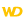• End of drawing
• Drawing in PHP
• Drawing in Browser code
WINDEVWEBDEVWINDEV MobileOthers<picLayer variable>.Rotation (Function)
In french: <Variable picCalque>.Rotation
Rotates an image in a variable of type picLayer.Example
MyWDPicImage is WDPic = "Test.gif"
MyLayer is picLayer

// Rotate layer by 45 degrees
Result1 is boolean
Result1 = MyLayer.Rotation(45)
IF Result1 = False THEN Error("The operation failed")
IMG_MyDrawing = MyWDPicImage
Syntax

Performing a simple rotation

<Result> = <picLayer image>.Rotation(<Rotation angle> [, <Options>])
<Result>: Boolean
• True if the rotation was performed,
• False otherwise.
<picLayer image>: picLayer variable
Name of the picLayer variable to be used.
<Rotation angle>: Real
Rotation angle (in degrees).
• If the angle is positive, the image rotates clockwise.
• If the angle is negative, the image rotates counterclockwise.
<Options>: Integer constantRotation options:
 drAdapt The image is flipped and enlarged. Then, its size is reduced to correspond to the initial image size. drDefault Enlarge the image (if necessary) so that it corresponds to the dimensions of rotated image.Constant used by default for the picLayer variables. drNoEnlarging The image is flipped but not enlarged: the image may be truncated.

Remarks

End of drawing

If <picLayer variable>.EndDrawing and <picLayer variable>.Rotation are called in the same process, the image changes will not be displayed.
Related Examples:Unit examples (WINDEV): Image rotation [ + ] Image rotation from any point that will be used as rotation center.
Component: wd280pnt.dll
Minimum version required
• Version 25